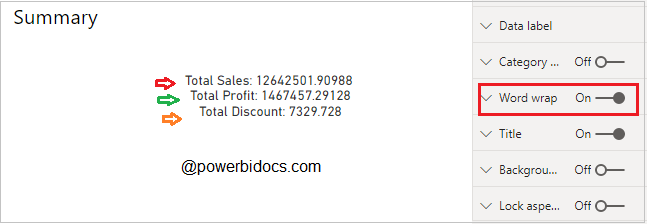Home » DAX » How to add line breaks in a string of text in DAX measure

# How to add line breaks in a string of text in DAX measureSuppose you want to display Total Sales, Total Profit & Total Discount values in single card visual as a string message.

So in order to achieve this you have to write some DAX code as given below and download dataset for this example-

#### DAX code-

Now create a measure & write below DAX code, after that drag this measure to card visual.

```Summary =
Var TotalSales = SUM('Global-Superstore'[Sales])
Var TotalProfit = SUM('Global-Superstore'[Profit])
Var TotalDiscount= SUM('Global-Superstore'[Discount])
Return
"Total Sales: " & TotalSales
& " Total Profit: " & TotalProfit
& " Total Discount: " & TotalDiscount```

#### See the outputCard Visual String Text

As you can see in above screenshot, it is showing all string text as in single line, but you want to see each text values as in new line for better visibility & easy to read.

#### Let’s see how you can make this possible-

• UNICHAR(10) DAX help us to add new line between string text.
• Turn on Word wrap for visual.

Now write below DAX code-

```Summary =
Var TotalSales = SUM('Global-Superstore'[Sales])
Var TotalProfit = SUM('Global-Superstore'[Profit])
Var TotalDiscount= SUM('Global-Superstore'[Discount])
Return
"Total Sales: " & TotalSales
& UNICHAR ( 10 ) & " Total Profit: " & TotalProfit
& UNICHAR ( 10 ) & " Total Discount: " & TotalDiscount```

#### See the final output with new line-UNICAHR DAX

Refer more DAX – DAX Tutorials

Hope you enjoyed the post. Your valuable feedback, question, or comments about this post are always welcome or you can leave us message on our contact form , we will revert to you asap.

## 2 thoughts on “How to add line breaks in a string of text in DAX measure”

1.doesnt work in multi card visual sorry

1.It’s work on Card visual only, not multi card